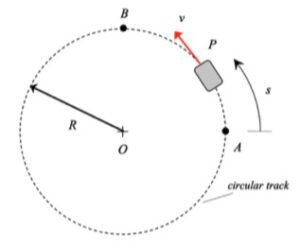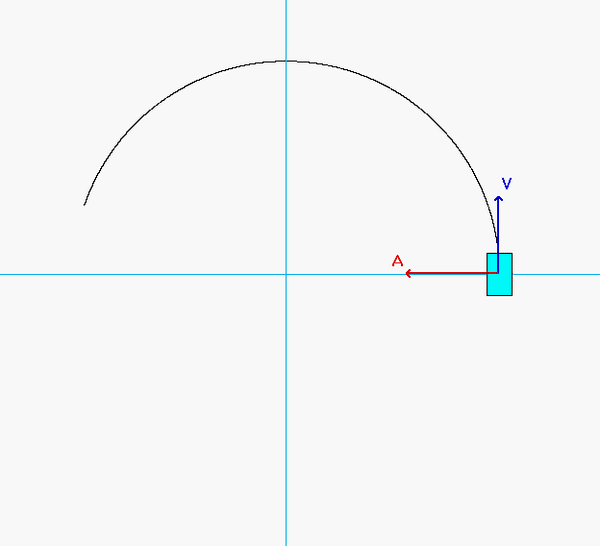# Homework H1.C - Sp23

[/embed]

 Problem statement Solution videoDISCUSSION and HINTS

As expected, the acceleration of P has both non-zero tangential and normal components.

• From the equation provided for speed as a function of distance traveled, the speed of P is monotonically decreasing over the range of motion shown in the animation below. Therefore, the tangential component of acceleration always points "backward" of the direction of motion.
• The normal component decreases as P moves along the track since the speed of P is decreasing.

Can you see these two things in the animation below?Recall that the general path description velocity and acceleration equations are given by the following:

v = v*et
a = v_dot*et + (v2/ρ)*en

Note that v_dot = dv/dt. For this problem, we do NOT know the speed as a function of time; instead, we know speed as a function of position, s. To find v_dot, we need to use the chain rule of differentiation:  dv/dt = (dv/ds)*(ds/dt) = v*(dv/ds).

## 34 thoughts on “Homework H1.C - Sp23”

1.Henry Francis Hellmann says:

Are we supposed to find v_dot be differentiating the v(s) equation?

1.CMK says:

Yes, v_dot is found from the differentiation of v with respect to TIME, "t". Note that v is given as a function of "s". To find dv/dt, you need to use the chain rule: dv/dt = (dv/ds)*(ds/dt) = v*(dv/ds).

1.Matthew Elias Brunton says:

"dv/dt = (dv/ds)*(ds/dt) = v*(dv/ds)"

2.Matthew Elias Brunton says:

"dv/dt = (dv/ds)*(ds/dt) = v*(dv/ds)"... In this, what is the final v? is it v_a or v_b? Or is it something else?

1.CMK says:

The v(s) given is the speed for any value of s > 0 (that is, for any position beyond point A).

The "final" v is v_B.

1.Connor Thomas Gockel says:

I think Matthew means the v in "v*(dv/ds)"

2.Matthew Issac Boxberger says:

Yes, but because the function is written in terms of s, you will need to use the chain rule, since v dot is differentiated by time.

2.Matthew Issac Boxberger says:

Should we use radians or degrees for the trigonometric equations in this problem?

1.CMK says:

Since b*s = dimensionless, the argument for cosine must be in radians.

2.Liam Quentin Johnson says:

3.Luis Martinez says:

After doing the differentiation mentioned above I believe we would get an expression for v_dot which we plug in the s value at positon b. I was wondering if it is correct to say this expression is also an expression in terms of s.

1.Anna Grace LiHua Hook says:

I believe this would be an expression in terms of s since all other variables are known/given and s is the only unknown.

4.Allie says:

When trying to find the values at point B, do we say that "s" in cos(bs) is 1/4 of the circumference since that will cancel with the units of b? Or is that not allowed because that isn't technically an angle?

1.John D Dec says:

Yes, we can express "s" in terms of the circumference which is dependent upon "R". I believe it is fine to do this as you know that the particle has moved one-fourth across the circle.

5.Madeline B says:

What does e^t stand for and how does it affect our equation?

1.John Reilly Kaufmann says:

e^t is the component of movement or acceleration that acts tangential to the object. e^n is the component that acts normal, or perpendicular to the motion of an object.

6.CMK says:

e^t is a unit vector (vector of length "1") that is tangent to the path. Similarly, e^n is a unit vector that is normal (perpendicular) to the path. You are more familiar with the orthogonal unit vectors i_hat and j_hat that are used in the Cartesian description for kinematics. e^t and e^n are the unit vectors that are used in the path description for kinematics.

1.Madeline B says:

Oh that makes sense. Thank you!

7.Madeline B says:

When calculating v_dot, do we need to have the s value solved for at B? I am confused on how to get the s value for the equation.

1.CMK says:

"s" is the distance traveled between points A and B. The question, therefore, is: what is the arc length of a quarter circle having a radius of R?

1.Madeline B says:

That's where I am a bit stumped. Do we just take 90 deg and put it into radians? Or are we suppose to use the sin equation that is given to us?

1.Madeline B says:

Cos equation derived into sin*

2.CMK says:

Recall that the arc length of a full circle (its circumference) is 2*pi*R. For a quarter-circle, the arc length is (1/4) of that amount.

1.Madeline B says:

Oh, my bad. I see. So technically speaking, we after we calculate v_dot, we can use that s value from arc length to get a scalar value for v_dot at B, which we can then use to get the acceleration at B?

1.CMK says:

Yes, that is correct.

1.Madeline B says:

But will we use v_A in that acceleration problem, or do we need to solve for a v_B?

1.CMK says:

I am not sure of your question. The speed of the automobile at ANY location on the path for s > 0 (which includes point B) depends of the speed of the automobile at point A through the equation: v = v_A*cos(bs). You are given the speed of the automobile at A; you will use that to find the speed and the rate of change of speed of the automobile when it is at B.

1.Madeline B says:

Sorry, you answered it! I was trying to figure out the speed at v_B, which I used at s with the equation given.

One more question, if you don't mind. When taking the magnitude of acceleration, we keep it in terms of e_t and e_n as our x^ and y^, right? So the magnitude would be the sqrt(e_t^2 + e_n^2)?

1.CMK says:

Yes, the magnitude of acceleration is sqrt(a_t^2 + a_n^2).

8.Kenneth James Robinette says:

In the acceleration equation (v_dot*et + (v^2/p)*en) I am confused about taking the time derivative of the velocity function given. There is no explicit t term in this equation so does the whole equation act as a constant and the derivative is equivalent to 0 or is the a term (like s) that can be evaluated in terms of time? If it is the later case would you do that?

1.CMK says:

As mentioned in the above discussion, since v is an explicit function of "s" instead of being an explicit function of "t", then you need to use the CHAIN RULE of differentiation to find dv/dt:
v_dot = dv/dt = (dv/ds)*(ds/dt) = v*(dv/ds).

2.Anna Grace LiHua Hook says:

Since the velocity equation V is in terms of S you have to do a similar process to the first homework where you are ultimately trying to get the derivative with respect to time. Since S is in terms of time you can use chain rule to take dv/ds * ds/dt and now the velocity equation derivative is with respect to t.

9.Andrew says:

How do we check our answers for feasibility and correctness?

1.Liam Quentin Johnson says:

I would try checking if your units are correct for the v_dot_B calculation and that you got a negative number (because the driver hits the breaks), if you used the chain rule incorrectly you may have ended up with something other than m/s^2 or get a positive result. You could also convert v_B to mph to check that it is a fairly realistic speed for a car.